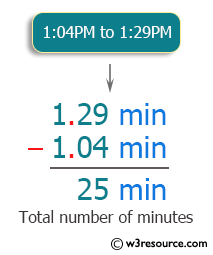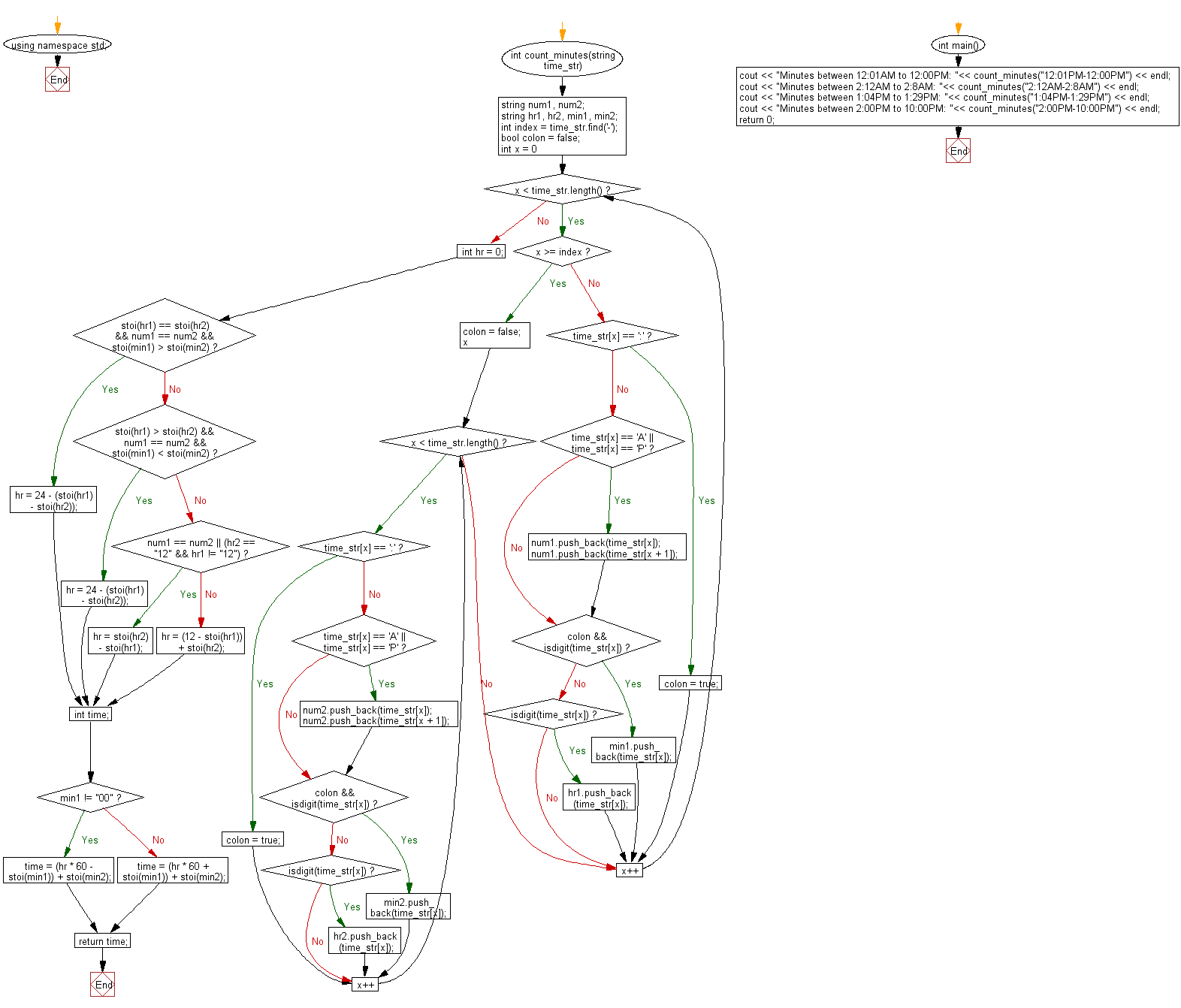﻿ C++: Find the total number of minutes between two times

# C++ Exercises: Find the total number of minutes between two given times

## C++ Basic: Exercise-85 with Solution

Write a C++ program to find the total number of minutes between two given times (formatted with a colon and am or pm).

Pictorial Presentation:Sample Solution:

C++ Code :

``````#include <iostream>
#include <string>
#include <sstream>
using namespace std;

int count_minutes(string time_str) {

string num1, num2;
string hr1, hr2, min1, min2;
int index = time_str.find('-');
bool colon = false;

for (int x = 0; x < time_str.length(); x++)
{
if (x >= index)
{
colon = false;
for (x; x < time_str.length(); x++)
{
if (time_str[x] == ':')
{
colon = true;
continue;
}

if (time_str[x] == 'A' || time_str[x] == 'P')
{
num2.push_back(time_str[x]);
num2.push_back(time_str[x + 1]);
}

if (colon && isdigit(time_str[x]))
{
min2.push_back(time_str[x]);
}
else if (isdigit(time_str[x]))
{
hr2.push_back(time_str[x]);
}
}
}
else
{
if (time_str[x] == ':')
{
colon = true;
continue;
}

if (time_str[x] == 'A' || time_str[x] == 'P')
{
num1.push_back(time_str[x]);
num1.push_back(time_str[x + 1]);
}

if (colon && isdigit(time_str[x]))
{
min1.push_back(time_str[x]);
}
else if (isdigit(time_str[x]))
{
hr1.push_back(time_str[x]);
}
}
}

int hr = 0;
if (stoi(hr1) == stoi(hr2) && num1 == num2 && stoi(min1) > stoi(min2))
{
hr = 24 - (stoi(hr1) - stoi(hr2));
}
else if (stoi(hr1) > stoi(hr2) && num1 == num2 && stoi(min1) < stoi(min2))
{
hr = 24 - (stoi(hr1) - stoi(hr2));
}
else if (num1 == num2 || (hr2 == "12" && hr1 != "12"))
{
hr = stoi(hr2) - stoi(hr1);
}
else
{
hr = (12 - stoi(hr1)) + stoi(hr2);
}

int time;
if (min1 != "00")
{
time = (hr * 60 - stoi(min1)) + stoi(min2);
}
else
{
time = (hr * 60 + stoi(min1)) + stoi(min2);
}

return time;
}

int main() {

cout << "Minutes between 12:01AM to 12:00PM: "<< count_minutes("12:01PM-12:00PM") << endl;
cout << "Minutes between 2:12AM to 2:8AM: "<< count_minutes("2:12AM-2:8AM") << endl;
cout << "Minutes between 1:04PM to 1:29PM: "<< count_minutes("1:04PM-1:29PM") << endl;
cout << "Minutes between 2:00PM to 10:00PM: "<< count_minutes("2:00PM-10:00PM") << endl;
return 0;
}
``````

Sample Output:

```Minutes between 12:01AM to 12:00PM: 1439
Minutes between 2:12AM to 2:8AM: 1436
Minutes between 1:04PM to 1:29PM: 25
Minutes between 2:00PM to 10:00PM: 480
```

Flowchart:C++ Code Editor:

Previous C++ Exercise: Convert a given number into hours and minutes.

Next C++ Exercise: Add up all the digits between two numbers .

What is the difficulty level of this exercise?

﻿

## C++ Programming: Tips of the Day

Why is there no std::stou?

The most pat answer would be that the C library has no corresponding "strtou", and the C++11 string functions are all just thinly veiled wrappers around the C library functions: The std::sto* functions mirror strto*, and the std::to_string functions use sprintf.

Ref: https://bit.ly/3wtz2qA

We are closing our Disqus commenting system for some maintenanace issues. You may write to us at reach[at]yahoo[dot]com or visit us at Facebook